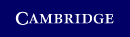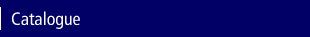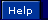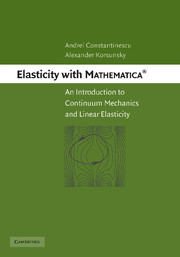Home > Catalogue > Elasticity with Mathematica ®## Resources and solutions

This title has free online support material available.

## Details

• Page extent: 0 pages

### Adobe eBook Reader

(ISBN-13: 9780511352898)

• Also available in Paperback | Hardback
• ### For price and ordering options, inspection copy requests, and reading lists please select:

Please note local prices may apply

This book introduces key ideas and principles in the theory of elasticity with the help of symbolic computation. Differential and integral operators on vector and tensor fields of displacements, strains and stresses are considered on a consistent and rigorous basis with respect to curvilinear orthogonal coordinate systems. As a consequence, vector and tensor objects can be manipulated readily, and fundamental concepts can be illustrated and problems solved with ease. The method is illustrated using a variety of plane and three-dimensional elastic problems. General theorems, fundamental solutions, displacements and stress potentials are presented and discussed. The Rayleigh-Ritz method for obtaining approximate solutions is introduced for elastostatic and spectral analysis problems. Containing more than 60 exercises and solutions in the form of Mathematica notebooks that accompany every chapter, the reader can learn and master the techniques while applying them to a large range of practical and fundamental problems.

• Contains Mathematica recipes for the solution of diverse elasticity problems • Includes a list of Mathematica tricks and tools for stress and strain analysis • Presents transparent explanations and illustrations of central concepts in elasticity using tensor calculus achieved by means of symbolic computation

### Contents

Preface; 1. Kinematics: displacements and strains; 2. Dynamics and statics: stresses and equilibrium; 3. Linear elasticity; 4. General principles in problems of elasticity; 5. Stress functions; 6. Displacement potentials; 7. Energy principles and variational formulations; Appendix 1. Differential operators; Appendix 2. Mathematica tricks; Appendix 3. Plotting parametric meshes; Bibliography; Index.

### Review

Review of the hardback: '… a useful book for anybody interested in problems in elasticity … provides a refreshing alternative to throwing every problem into a finite element solver. It would be an excellent textbook for a graduate course in elasticity.' Contemporary Physics